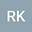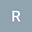A method for writing a number as a binary quadratic form
••• Robert Kimera,
• robkim55
Robert Kimera

Corresponding Author:robkim55@gmail.com

Author Profile## Abstract

An algorithm  to find a number T given a particular binary quadratic form.
Given a number T the question is whether we can write in a binary quadratic form.
$$Let\ T=Ax^2+Bxy+Cy^2$$ then ; How can we write T in terms of the coefficients A, B and C if we specify that all the letters involved must be integers  T,A, B,C, x,y $$T,A,B,C,x,y\ \ belong\ in\ Z$$
Let us first give an example.  Suppose we have 17 as the number we want to write down. We can write it as                     $$17=x^2+y^2=4^2+1^2$$;   and if we want to write the number 43, we can write it thus;  $$43=6^2+6.1+1^2=x^2+xy+y^2$$.
Then the question of representation arises; how can we write any number as a binary quadratic form?  Given that  $$Ax^2+Bxy+Cy^2-T=0$$, find  general expressions of numbers A, B, C, T such that x and y are integers.  In other words given an integer T find the way it can be represented by  A, B, C.
Proposition:
If  $$A=\left(PM-pm\right)$$          $$B=\left\{\left(PN+QM\right)-\left(pn+qm\right)\right\}$$ $$C=\left(QN-qn\right)$$, then
$$T=\frac{\left(M+N-m-n\right)c_1+\left(P+Q-p+q\right)}{m+n}$$ with    $$m+n=1;$$ $$Pq-pQ=1$$
Proof:
Let us write  $$\left(Ax^2+Bxy+Cy^2-T\right)=GH-JK=0\ \ \ \ \ \ \ \ \ \left(1\right)$$
where $$G=\left(Px+Qy+c_1\right)\ \ \ \ \ \ \ \ \ \ \ \ \ \ \ \ \ \ \ \ \ \ \ \ \ \ \ \left(2\right)\$$
and        $$H=\left(Mx+Ny+c_2\right)\ \ \ \ \ \ \ \ \ \ \ \ \ \ \ \ \ \ \ \ \ \left(3\right)$$
and        $$J=\left(px+qy+c_3\right)\ \ \ \ \ \ \ \ \ \ \ \ \ \ \ \ \ \ \ \ \ \ \ \ \ \ \left(4\right)$$
and         $$K=\left(mx+ny+c_4\right)\ \ \ \ \ \ \ \ \ \ \ \ \ \ \ \ \ \ \ \ \ \ \ \left(5\right)$$
Now we expand  the expression   $$GH-JK=0\ \ \ or\ \ \left(2\right)\left(3\right)-\left(4\right)\left(5\right)=0$$ in the above equations.
$$\left(Px+Qy+c_1\right)\left(Mx+Ny+c_2\right)-\left(px+qy+c_3\right)\left(mx+ny+c_4\right)=0\ \ \left(6\ \ \right)$$
Expanding  the above expression (6) and gathering all terms as powers of x and y;
$$\left(PM-pm\right)x^2=Ax^2\ \ \ \ \ \ \ \ \ \ \ \ \left(7\right)$$
$$\left\{\left(PN+QM\right)-\left(pm-qn\right)\right\}xy=Bxy\ \ \ \ \ \ \ \left(8\right)$$
$$\left(QN-qn\right)y^2=Cy^2\ \ \ \ \ \ \ \ \ \ \ \ \ \ \left(9\right)$$
$$\left\{\left(Qc_2+Nc_1\right)-\left(qc_4+nc_3\right)\right\}y=Dy=0y\ \ \ \ \ \ \left(10\right)$$
$$\left\{\left(Pc_2+Mc_1\right)-\left(pc_4+mc_3\right)\right\}x=Ex=0x\ \ \ \ \ \left(11\right)$$
$$c_1c_3-c_2c_4=-T\ \ \ \ \ \ \ \ \ \ \left(12\right)$$
We want to introduce conditions  for integer solutions;
We say that           $$Pq-pQ=+1$$
This is derived from  the two equations ;
$$Px+Qy+c_1=0\ \ \ \ \ \ \ \ \ \ \ \ \ \ \ \ \ \ \ \ \left(14\right)$$
$$px+qy+c_3=0\ \ \ \ \ \ \ \ \ \ \ \ \ \ \ \ \ \ \ \ \ \ \ \left(15\right)$$
$$GH-JK=0H-0K=0\ \ \ \ \ \ \ \ \ \ \left(16\right)$$
Notice that the choice of (14) and (15) make the expression  zero.
$$c_2-c_1=1,\ \ \ \ \ therefore\ \ c_3-c_1=-T$$
We add  equation (10)  to (11) and simplify;
$$\left\{\left(\left[P+Q\right]c_2+\left[M+N\right]c_1-\left(\left[p+q\right]c_4+\left[m+n\right]c_3\right)\right)\right\}=D+E=0$$
Replacing c3, i.e.   $$c_3=c_1-T$$,  we have:
$$\left[\left\{\left(P+Q\right)-\left(p+q\right)\right\}+\left\{\left(M+N\right)c_1-\left(m+n\right)\left(c_1-T\right)\right\}\right]=0$$
Solving for  T  in the above     equation we have;   $$T=\frac{\left(M+N-m-n\right)c_1+\left(P+Q-p-q\right)}{m+n}$$.
Notice that   $$c_1$$    is a free variable free to take on any integer value . So the above  expression is an expression of any integer T.
Algorithm
This suggest an algorithm for finding a number T given a particular   binary quadratic form.
1 Write down the binary quadratic equation as the difference of two products GH-JK=0.
2. Set (m+n)=1. This is to ensure that T is an integer rather than a fraction.
3. Set (Pq-pQ)=1. This will ensure that the solution (x,y) is made up of integers x and y.
4. Set [(M+N)-(m+n)] a small integer (but not always).
5. Then find $$c_1$$ from the equation  $$T=c_1Q_1+R$$
6. Then use P,Q,M,N, m,n,p,q, to find A, B, C.